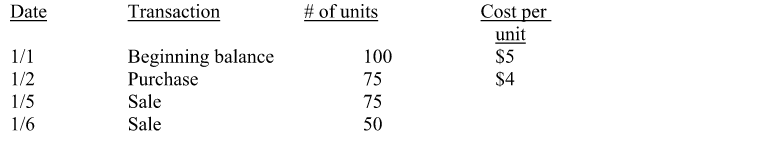# Problem: Maxell Company uses the FIFO method to value inventory and had the following transactions in the period. What are Maxell’s cost of goods sold and ending inventory balance in dollars for the period? a. COGS - $625; Ending Inventory -$175 b. COGS - $575; Ending Inventory -$225 c. COGS - $550; Ending Inventory -$250 d. COGS - $600; Ending Inventory -$200 e. None of the above is correct.

###### Problem Details

Maxell Company uses the FIFO method to value inventory and had the following transactions in the period.

What are Maxell’s cost of goods sold and ending inventory balance in dollars for the period?

a. COGS - $625; Ending Inventory -$175

b. COGS - $575; Ending Inventory -$225

c. COGS - $550; Ending Inventory -$250

d. COGS - $600; Ending Inventory -$200

e. None of the above is correct.Frequently Asked Questions

What scientific concept do you need to know in order to solve this problem?

Our tutors have indicated that to solve this problem you will need to apply the Periodic Inventory - FIFO, LIFO, and Average Cost concept. You can view video lessons to learn Periodic Inventory - FIFO, LIFO, and Average Cost. Or if you need more Periodic Inventory - FIFO, LIFO, and Average Cost practice, you can also practice Periodic Inventory - FIFO, LIFO, and Average Cost practice problems.

What is the difficulty of this problem?

Our tutors rated the difficulty ofMaxell Company uses the FIFO method to value inventory and h...as medium difficulty.